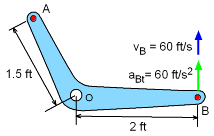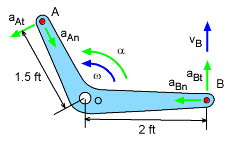Ch 5. Rigid Body General Motion Multimedia Engineering Dynamics Fixed Axis Rotation Plane Motion Velocities Zero Velocity Point Plane Motion Accelerations Multiple Gears Rot. Coord. Velocities Rot. Coord. Acceleration
 Chapter - Particle - 1. General Motion 2. Force & Accel. 3. Energy 4. Momentum - Rigid Body - 5. General Motion 6. Force & Accel. 7. Energy 8. Momentum 9. 3-D Motion 10. Vibrations Appendix Basic Math Units Basic Equations Sections Search eBooks Dynamics Fluids Math Mechanics Statics Thermodynamics Author(s): Kurt Gramoll ©Kurt GramollDYNAMICS - EXAMPLERotating Bracket Example A angled bracket rotates about a fixed shaft O. If the tangential acceleration and velocity of point B is 400 ft/s2 and 60 ft/s, respectively, what is the total acceleration magnitude of point A? SolutionAngular Velocity and Acceleration This problem is a basic fixed-axis rotation problem since the problem explicitly states there is a fixed shaft. First, determine the angular velocity and angular acceleration. Angular Velocity      vB = rB ω      60 = 2 ω      ω = 30 rad/s Angular Acceleration      aBt = rB α      400 = 2 α      α = 200 rad/s2 Use ω and α to find normal and tangent acceleration of point A      aAt = rA α            = (1.5)(200) = 300 ft/s2      aAn = ω2rA            = 900 (1.5) = 1,350 ft/s2 Total Acceleration      aA = (1,3502 + 3002)0.5 = 1,383 ft/s2 A = 1,383 ft/s2

Practice Homework and Test problems now available in the 'Eng Dynamics' mobile app
Includes over 400 problems with complete detailed solutions.
Available now at the Google Play Store and Apple App Store.